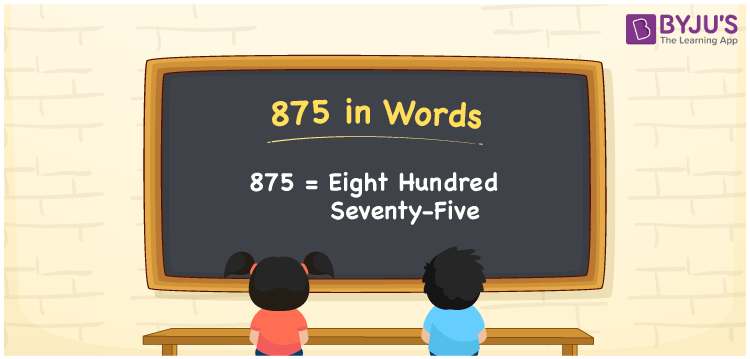# 875 in Words

We can write 875 in words as Eight hundred seventy-five. If you bought 875 candles to light for a festival, then you can say, “I bought Eight hundred seventy-five candles for the festival”. Therefore, we use the number name of 875 to communicate the numerical quantity to others. Let’s learn how to derive the word form of 875 with the help of a place value chart here in this article.

 875 in words Eight hundred seventy-five Eight hundred seventy-five in Numbers 875

## 875 in English Words

We generally use the English alphabet to write numbers in words. Thus, we spell 875 in English words as “Eight hundred seventy-five”.## How to Write 875 in Words?

As we can see, 875 is a three-digit number so let’s make a place value chart with three columns, as shown below.

 Hundreds Tens Ones 8 7 5

Here, ones = 5, tens = 7, hundreds = 8

By expanding the above numbers, we get;

8 × Hundred + 7 × Ten + 5 × One

= 8 × 100 + 7 × 10 + 5 × 1

= 800 + 70 + 5

= Eight hundred + Seventy + Five

= Eight hundred seventy-five

Thus, 875 in words is written as Eight hundred seventy-five.

Learn in detail about place value here.

875 is a natural number that precedes 876 and succeeds 874.

875 in words – Eight hundred seventy-five

Is 875 an even number? – No

Is 875 an odd number? – Yes

Is 875 a prime number? – No

Is 875 a composite number? – Yes

Is 875 a perfect square number? – No

Is 875 a perfect cube number? – No

## Frequently Asked Questions on 875 in Words

Q1

### How do you write 875 in word form?

The word form of the number 875 is “Eight hundred seventy-five”.
Q2

### Express 875.875 in words.

We can express the decimal number 875.875 in words as “Eight hundred seventy-five point eight seven five”.
Q3

### How to write Rs. 875 in words on a cheque?

On a cheque, an amount of Rs. 875 can be written in words as “Eight hundred seventy-five rupees only”.